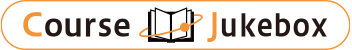### CourseDetailDegree
Bachelor
Course delivery methods
face-to-face
Subject
Engineering & technology
Program
School
College of Engineering
Department
Campus
Main Campus
Classroom
Course Offering Year
Course Offering Month
February - June
Weekday and Period
Tuesday 3,4,5
Capacity
40
Credits
3
Language
English
Course Number
ME5141 (522EU2960)

### Computational Fluid Mechanics National Taiwan University

#### Course Overview

This is an introductory course to computational methods for fluid dynamics. Following a preface to numerical simulation and a review of the governing equations for mass, momentum, and energy, the structure and mathematical behaviors of partial differential equations will be discussed, which are classified as hyperbolic, parabolic, and elliptic types. A discretization scheme to approximate the mathematical models, the finite-difference method, is described along with the analyses for the resulting errors and stability, followed by strategies of allocation and transformation of grids. Some simple CFD techniques will then be illustrated, in terms of various schemes suited for different categories of PDE's. Various methods of discretization other than the finite-difference approach, such as finite-volume method and finite-element method, shall be briefly mentioned if time is available.

#### Learning Achievement

Part I: Fundamentals of mathematical and physical models 1.Philosophy of computational fluid dynamics(83dc) 2.The governing equations for fluid dynamics(83dc) 3.Mathematical behavior of partial differential equations Part II: Numerical approaches 4.Basic aspects of discretization 5.Grids with appropriate transformations 6.Numerical methods for hyperbolic PDE: wave equation 7.Numerical methods for parabolic PDE: heat equation(83dc) 8.Numerical methods for elliptic PDE: Laplace's equations

Kuo-Long Pan

#### Other information

(College of Engineering) Graduate Institute of Mechanical Engineering,
(College of Engineering) Department of Mechanical Engineering